# RD Sharma Class 8 Solutions Chapter 9 Linear Equations in One Variable Ex 9.1

In this chapter, we provide RD Sharma Class 8 Solutions Chapter 9 Linear Equations in One Variable Ex 9.1 for English medium students, Which will very helpful for every student in their exams. Students can download the latest RD Sharma Class 8 Solutions Chapter 9 Linear Equations in One Variable Ex 9.1 Maths pdf, free RD Sharma Class 8 Solutions Chapter 9 Linear Equations in One Variable Ex 9.1 book pdf download. Now you will get step by step solution to each question.

### RD Sharma Solutions for Class 8 Chapter 9 Linear Equations in One Variable Ex 9.1Download PDF

Solve each of the following equations and also verify your solution :
Question 1.
914 = y – 113
Solution: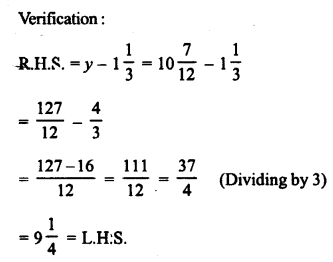Question 2.
5×3 + 25 = 1
Solution: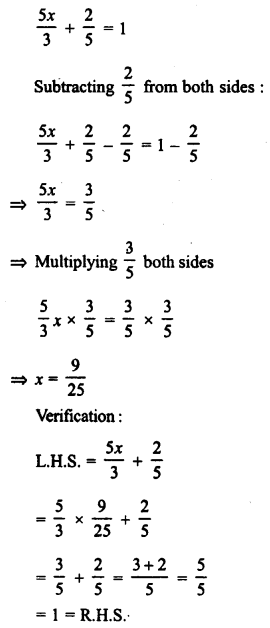Question 3.
x2 + x3 + x4 = 13
Solution:Question 4.
x2 + x8 = 18
Solution: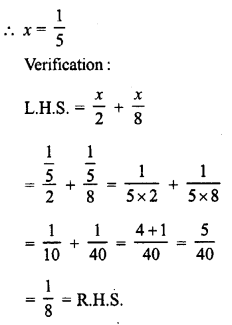Question 5.
2×3 – 3×8 = 712
Solution:Question 6.
(x + 2) (x + 3) + (x – 3) (x – 2) – 2x (x + 1) = 0
Solution:
(x + 2) (x + 3) + (x – 3) (x – 2) – 2x (x + 1) = 0
⇒ [x² + (2 + 3) x + 2 x 3] + [x² + (-3 – 2) x + (-3) (-2)] – 2x² – 2x = 0
⇒ x² + 5x + 6 + x² – 5x + 6 – 2x² – 2x = 0
⇒ x² + x² – 2x² + 5x – 5x – 2x + 6 + 6 = 0
⇒ -2x + 12 = 0
Subtracting 12 from both sides,
-2x + 12 – 12 = 0 – 12
⇒ -2x = -12
Dividing by -2,
x = 6
Verification:
L.H.S. = (x + 2) (x + 3) + (x – 3) (x – 2) – 2x (x + 1)
= (6 + 2) (6 + 3) + (6 – 3) (6 – 2) – 2 x 6 (6 + 1)
= 8 x 9 + 3 x 4 – 12 x 7
= 72 + 12 – 84
= 84 – 84
= 0
= R.H.S.

Question 7.
x2 – 45 + x5 +3×10 = 15
Solution: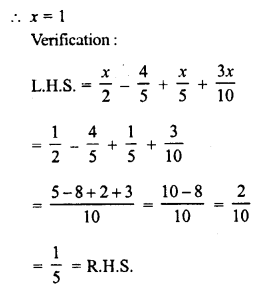Question 8.
7x + 35 = 110
Solution:Question 9.
2x–13 – 6x–25 = 13
Solution:Question 10.
13 (y – 4) – 3 (y – 9) – 5 (y + 4) = 0
Solution:
13 (y – 4) – 3 (y – 9) – 5 (y + 4) = 0
⇒ 13y – 52 – 3y + 27 – 5y – 20 = 0
⇒ 13y – 3y – 5y – 52 + 27 – 20 = 0
⇒ 13y – 8y – 72 + 27 = 0
⇒ 5y – 45 = 0
Dividing by 5,
y = 9
Verification:
L.H.S. = 13 (y – 4) – 3 (y – 9) – 5 (y + 4)
= 13 (9 – 4) – 3 (9 – 9) – 5 (9 + 4)
= 13 x 5 – 3 x 0 – 5 x 13
= 65 – 0 – 65
= 0
= R.H.S.

Question 11.
23 (x – 5) – 14 (x – 2) = 92
Solution: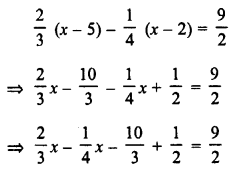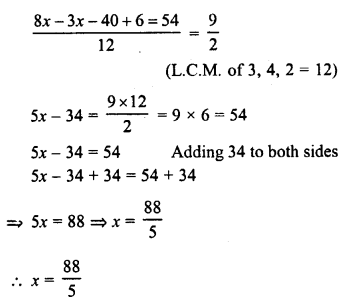All Chapter RD Sharma Solutions For Class 8 Maths

—————————————————————————–

All Subject NCERT Exemplar Problems Solutions For Class 8

All Subject NCERT Solutions For Class 8

*************************************************

I think you got complete solutions for this chapter. If You have any queries regarding this chapter, please comment on the below section our subject teacher will answer you. We tried our best to give complete solutions so you got good marks in your exam.

If these solutions have helped you, you can also share rdsharmasolutions.in to your friends.